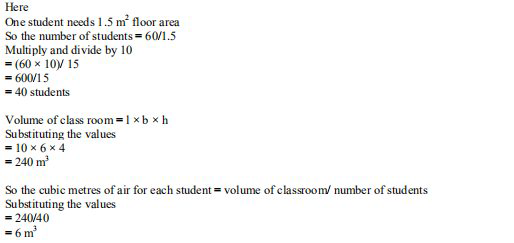# ML Aggarwal Solutions Class 9 Mathematics Solutions for Mensuration Exercise 16.4 in Chapter 16 - Mensuration

A classroom is 10 m long, 6 m broad and 4 m high. How many students can it accommodate if one

student needs 1.5 \mathrm{m}^{2} of floor area? How many cubic metres of air will each student have?

The given dimensions of the classroom are

Length (l) = 10 m

Height (h) = 4 m

We know that

The floor area of classroom = l × b

Substituting the values

= 10 × 6

=60 \mathrm{m}^{2}Related Questions

Lido

Courses

Teachers

Book a Demo with us

Syllabus

Maths
CBSE
Maths
ICSE
Science
CBSE

Science
ICSE
English
CBSE
English
ICSE
Coding

Terms & Policies

Selina Question Bank

Maths
Physics
Biology

Allied Question Bank

Chemistry
Connect with us on social media!